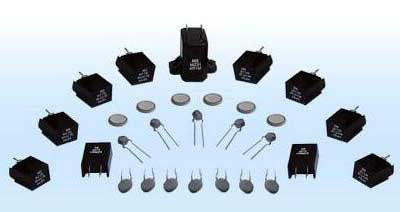﻿ Ptc thermistor parameters_

China sensor manufacturers

# Ptc thermistor parameters

1, rated zero power resistor R25
Zero power resistor. When the PTC thermistor value is measured at a certain temperature, the power consumption added to the PTC thermistor is extremely low, so that the resistance change of the PTC thermistor caused by its power consumption is negligible. The rated zero power resistance refers to the zero power resistance measured at an ambient temperature of 25 °C. The minimum resistance Rmin refers to the minimum zero power resistance value that a PTC thermistor can have.

2, Curie temperature Tc
For PTC thermistor applications, the temperature at which the resistance value begins to increase steeply is important, which we define as the Curie temperature. The resistance of the PTC thermistor corresponding to the Curie temperature RTc = 2*Rmin.

3, temperature coefficient α
The temperature coefficient of a PTC thermistor is defined as the relative change in resistance due to temperature changes. If the temperature coefficient is larger, the PTC thermistor is more sensitive to temperature changes.

4, surface temperature Tsurf
The surface temperature Tsurf refers to the temperature of the surface of the PTC thermistor when the PTC thermistor is in a state of thermal equilibrium with a predetermined voltage and with the surrounding environment for a long time.

5, the action current Ik
The current flowing through the PTC thermistor is sufficient to cause the PTC thermistor to rise above the Curie temperature, which is called the operating current. The minimum value of the operating current is called the minimum operating current.

6, action time ts
In the environment of 25 ° C, the PTC thermistor is added with an initial current (guaranteed to be the operating current), and the time elapsed when the current through the PTC thermistor is reduced to 50% of the starting current is the operating time.

7, no action current INk
The current flowing through the PTC thermistor is insufficient to cause the PTC thermistor to rise above the Curie temperature, which is called a non-operating current. The maximum value of the operating current is called the maximum non-operating current.

8, the maximum current Imax
The maximum current is the highest current withstand capability of the PTC thermistor. The PTC thermistor will fail when the maximum current is exceeded.

9, residual current Ir
The residual current is the current in the thermal equilibrium state at the maximum operating voltage Vmax.

10, the maximum working voltage Vmax
The maximum operating voltage is the maximum voltage that is allowed to remain continuously on the PTC thermistor at the specified ambient temperature. For the same product, the higher the ambient temperature, the lower the maximum operating voltage value.

11, rated voltage VN
The rated voltage is the supply voltage below the maximum operating voltage Vmax. Usually Vmax = VN + 15%

12, breakdown voltage VD
The breakdown voltage refers to the highest voltage withstand capability of the PTC thermistor. The PTC thermistor will be broken down above the breakdown voltage and cause failure.﻿
﻿